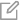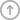# 谁才是维密大秀的宠儿？

NYCDSA

2018-12-03

DT数据侠与纽约数据科学院（New York City Data Science Acadamy）合作的第一期数据侠Python训练营10月结营，在完成对数据爬取、数据分析与数据可视化的训练之后，DT君选取了一些训练营成员的作品供大家欣赏。 这篇文章就来自于数据侠Haoyue Zhang，他通过抓取、可视化与分析了维密大秀的数据，发现了很多“维多利亚”的“秘密”哟！大家先睹为快吧!

• 谁是参加过最多次走秀的模特？
• 哪位模特开秀/闭秀/佩戴FantasyBra的次数最多？
• 维密天使都来自于哪些国家？
• 开秀/闭秀/佩戴FantasyBra来自于哪些国家？
• 成为维密天使与否与参加大秀的次数是否有关？
• 开秀/闭秀/佩戴FantasyBra与参加大秀的次数是否有关？
• 开秀/闭秀/佩戴FantasyBra的模特一定都是维密天使吗？
• 中国模特们在维密大秀是否也有了一席之地？
• 维密选人是不是越来越“花心”了？

## 数据爬取

1.记录了每年大秀的时间、地点、参加模特、演出者等信息的summary table

2.记录了每年Fantasy Bra的佩戴模特、模特国籍、bra价值的等信息的表格

3.记录了每年开秀、闭秀模特的表格

4.维密天使的信息表格

5.参加维密大秀的模特（不包括维密天使）的表格

Summary table的Spider代码如下:

from scrapy import Spider

from summary.items import SummaryItem

class SummarySpider(Spider):

name = 'summary_spider'

allowed_urls = ['https://en.wikipedia.org']

start_urls = ['https://en.wikipedia.org/wiki/Victoria\'s_Secret_Fashion_Show']

def parse(self, response):

rows = response.xpath('//*[@id="mw-content-text"]/div/table//tr')

event_patterns = ['./td/a/text()','./td/text()']

location_patterns = ['./td//a/text()','./td/text()']

for row in rows:

for event_pattern in event_patterns:

Event = row.xpath(event_pattern).extract_first()

if Event:

break

for location_pattern in location_patterns:

Locations = row.xpath(location_pattern).extract()

if Locations:

break

Models = row.xpath('./td/div/ul//a/text()').extract()

Performers = row.xpath('./td//a/text()').extract()

item = SummaryItem()

item['Event'] = Event

item['Locations'] = Locations

item['Models'] = Models

item['Performers'] = Performers

yield item

from scrapy import Spider

from fantasy.items import FantasyItem

class FantasySpider(Spider):

name = 'fantasy_spider'

allowed_urls = ['https://en.wikipedia.org']

start_urls = ['https://en.wikipedia.org/wiki/Victoria\'s_Secret_Fashion_Show']

def parse(self, response):

rows = response.xpath('//*[@id="mw-content-text"]/div/table//tr')

for row in rows:

year = row.xpath('./td/text()').extract_first()

name = row.xpath('./td/text()').extract_first()

model_name = row.xpath('./td/a/text()').extract()

model_country = row.xpath('./td/span/a/@title').extract()

value = row.xpath('./td/text()').extract()

in_show = row.xpath('./td/text()').extract_first()

item = FantasyItem()

item['year'] = year

item['name'] = name

item['model_name'] = model_name

item['model_country'] = model_country

item['value'] = value

item['in_show'] = in_show

yield item

from scrapy import Spider

from openclose.items import OpencloseItem

class OpencloseSpider(Spider):

name = 'openclose_spider'

allowed_urls = ['https://en.wikipedia.org']

start_urls = ['https://en.wikipedia.org/wiki/Victoria\'s_Secret_Fashion_Show']

def parse(self, response):

rows = response.xpath('//*[@id="mw-content-text"]/div/table//tr')

name_patterns = ['./td/a/text()', './td/a/text()']

country_patterns = ['./td/span/a/@title','./td/span/a/@title']

for row in rows:

for name_pattern in name_patterns:

model_name = row.xpath(name_pattern).extract_first()

if model_name:

break

for country_pattern in country_patterns:

model_country = row.xpath(country_pattern).extract_first()

if model_country:

break

item = OpencloseItem()

item['model_name'] = model_name

item['model_country'] = model_country

yield item

from scrapy import Spider

from ModelList.items import ModellistItem

class ModellistSpider(Spider):

name = 'modellist_spider'

allowed_urls = ['https://en.wikipedia.org']

start_urls = ['https://en.wikipedia.org/wiki/List_of_Victoria%27s_Secret_models']

def parse(self, response):

rows = response.xpath('//*[@id="mw-content-text"]/div/table/tbody//tr')

for row in rows:

model_name = row.xpath('./td//a/@title').extract_first()

model_country = row.xpath('./td//a/@title').extract_first()

item = ModellistItem()

item['model_name'] = model_name

item['model_country'] = model_country

yield item

from scrapy import Spider

from modellist2.items import Modellist2Item

class modellit2Spider(Spider):

name = 'modellist2_spider'

allowed_urls = ['https://en.wikipedia.org']

start_urls = ['https://en.wikipedia.org/wiki/List_of_Victoria%27s_Secret_models']

def parse(self, response):

rows = response.xpath('//*[@id="mw-content-text"]/div/table/tbody//tr')

for row in rows:

model_name = row.xpath('./td/span/a/text()').extract_first()

model_country = row.xpath('./td/a/@title').extract_first()

item = Modellist2Item()

item['model_name'] = model_name

item['model_country'] = model_country

yield item

1.Summary Table

2.开秀/闭秀记录

#编写for循环，查找历年走秀记录中的每一个（模特，年份）组合是否出现在开秀记录中

for i in range (0,777):

if (df_model_summary['Event_year'][i], df_model_summary['model_name'][i]) in [(df_open['year'][i], df_open['model_name'][i]) for i in range(0,22)]:

df_model_summary['open'][i] = 1

3.Fantasy Bra

2014年维密推出了一对fantasy bra，由两位模特佩戴，但是数据中只用一行记录了下来，在这里我们用之前拆分模特名字的方法处理一下，将2014年的记录分成两行，美味模特占一行，然后手动修正国籍、bra价值，使2014年的记录以如下形式呈现：

4.维密天使表格

5.参与走秀的非天使模特表格

for i in range (0,236):

if model_list_2.model_country[i] is np.nan:

model_list_2.model_country[i] = model_list_2.model_country[i-1]

1. 历年走秀记录（每一行是每年参与走秀的一位模特，记录模特在当年的大秀中是否开秀/闭秀/佩戴fb）
2. 全部模特列表（每一行是一位模特，记录该模特的国籍、是否为维密天使）

temp = pd.merge(df_show_count, df_model, how = 'left', on ='model_name')

temp.loc[temp.model_country.isnull(),:]

Q1. 谁是参加过最多次走秀的模特？

Q2. 哪位模特开秀/闭秀/佩戴Fantasy Bra的次数最多？

Q3. 维密天使都来自于哪些国家？

Q4. 开秀/闭秀/佩戴Fantasy Bra最多次的模特来自于哪些国家？

Q5. 成为维密天使与否与参加大秀的次数是否有关？

#跑一个2 sample t-test 验证我们的猜想

number_of_shows_walked = model_summary['number_of_shows_walked']

shows_non_angel = number_of_shows_walked[model_summary['angel']==0]

shows_angel = number_of_shows_walked[model_summary['angel']==1]

shows_non_angel = shows_non_angel.dropna()

shows_angel = shows_angel.dropna()

from scipy import stats

stats.ttest_ind(shows_non_angel ,shows_angel)

Ttest_indResult(statistic=-13.017789081684617, pvalue=2.3704995718195955e-30)

P值接近0，说明非天使模特和天使的走秀次数差异真的是非常大了！【不过这也不能说明走秀次数多就是成为天使的原因……有一些计量经济学基础的朋友都懂的

Q6. 开秀/闭秀/佩戴Fantasy Bra与参加大秀的次数是否有关？这些模特一定都是维密天使吗？

a.开秀

b.闭秀

1. Fantasy Bra

Q7. 中国模特们在维密大秀是否也有了一席之地？

Q8. 维密选人是不是越来越“花心”了？

year = list(range(1995, 2019))

#将每年走秀的所有模特名字放在一个set中，从1995年到2018年一共24个set

model_list_by_year = list(range(0,24))

for i in range(0,24):

model_list_by_year[i] = set(df_show['model_name'][df_show['Event_year']==i+1995])

#令每一年的模特名字和前一年的取并集

model_list_by_year_accumulative = list(range(0,24))

model_list_by_year_accumulative = model_list_by_year

for i in range (1,24):

model_list_by_year_accumulative[i] = list(model_list_by_year[i].union(model_list_by_year[i-1]))

#将年份和每年的accumulative模特名单放在一起，组成一个dataframe

model_set = pd.DataFrame({'year': year, 'model_set': model_list_by_year_accumulative})

#以1996年为例，先提取出某一年的走秀记录，到model_set中对年份进行匹配，再查找模特名字

model_1996 = df_show.loc[df_show['Event_year']==model_set['year']].apply(lambda x: x['model_name'] not in model_set['model_set'], axis = 1)

#写一个for循环，对每一年分别进行查询操作，list中每一年都对应一个boolean series，一共有23年，得到一个由23个series组成的list

new_model=list()

for i in range(0,23):

new_model.append(df_show.loc[df_show['Event_year']==model_set['year'][1+i]].apply(lambda x: x['model_name'] not in model_set['model_set'][i], axis = 1))

#将23个series合并成一整个series, 再转成datarame

new_model_list = new_model

for i in range(1,23):

new_model_list =  pd.concat([new_model_list,new_model[i]],axis=0)

new_model_list = new_model_list.to_frame()

new_model_list.columns=['new_model']

#取df_show从1996年开始的数据，研究启用新模特比例的问题

df_show_new = df_show.loc[df_show['Event_year']!=1995,:]

#和new_model变量合并

df_show_new = pd.concat([df_show_new, new_model_list],axis=1)

#计算每年新上秀的模特比例，画图

plt.figure(figsize=[12,6])

plt.xlabel('Year of the show')

plt.ylabel('Ratio of New Models')

(df_show_new.loc[df_show_new['new_model']==True,:].groupby('Event_year')['model_name'].count()/df_show_new.groupby('Event_year')['model_name'].count()).plot.bar(color= '#f08080')

plt.title('How many new models were chosen every year?', fontsize=15)

## 关于DT×NYCDSA

DT财经与纽约数据科学学院是战略合作伙伴。DT×NYCDSA 系合作开设的系列专栏。